# Python--matplotlib绘图

matplotlib是python最著名的绘图库，它提供了一整套和matlab相似的命令API，十分适合交互式地行制图。其中，matplotlib的pyplot模块一般是最常用的，可以方便用户快速绘制二维图表。

## 绘制一个最简单的折线图

import matplotlib.pyplot as plt

x = [1, 2, 3, 4]
y = [1.2, 2.5, 4.5, 7.3]

# plot函数作图
plt.plot(x, y)

# show函数展示出这个图，如果没有这行代码，则程序完成绘图，但看不到
plt.show()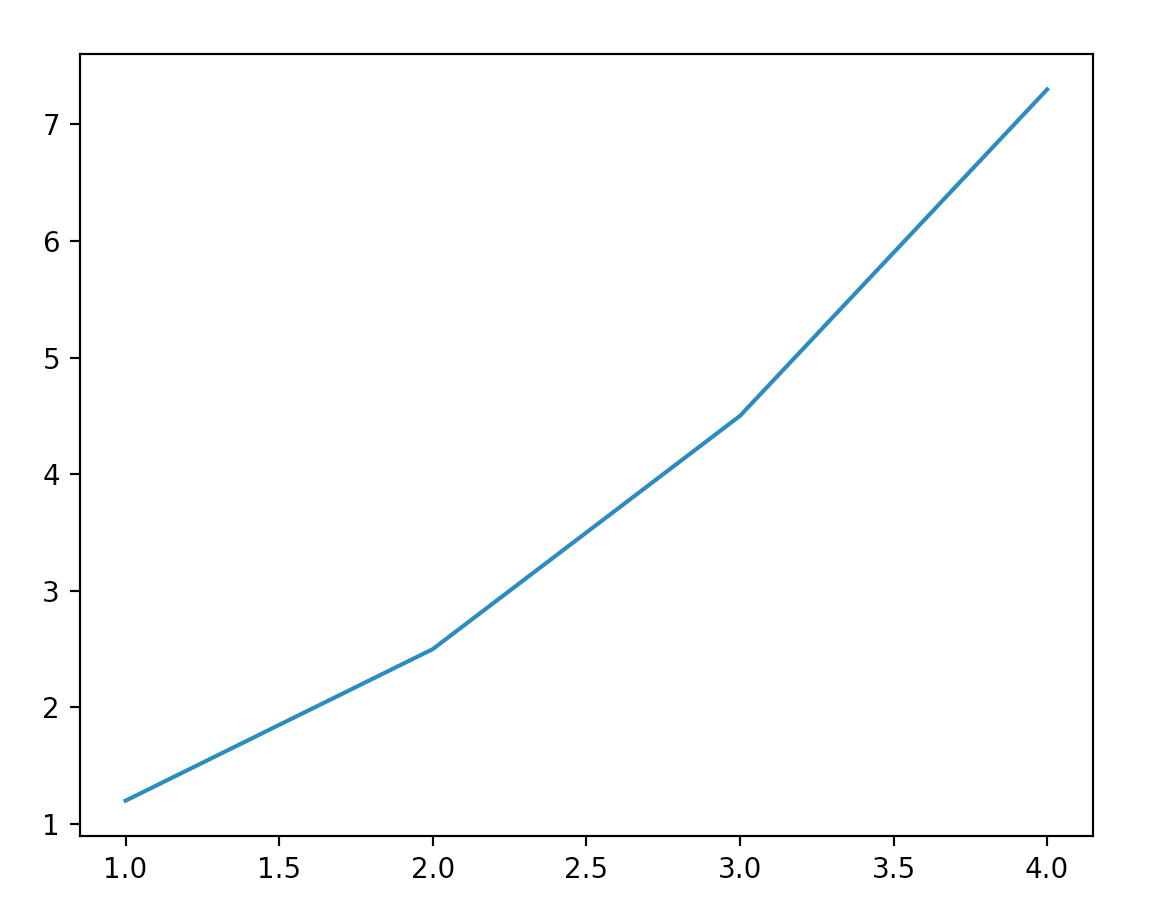plt.savefig("test.png", dpi=120)

## 绘制不同风格的线条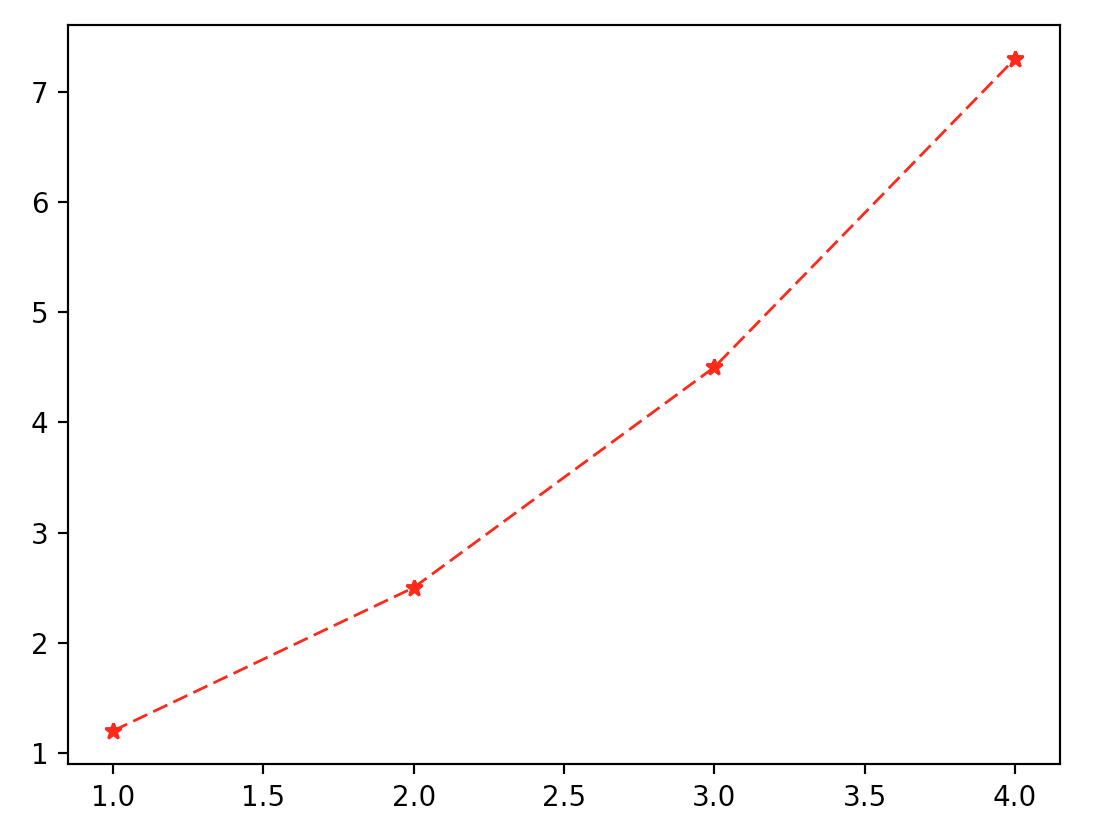import matplotlib.pyplot as plt

x = [1, 2, 3, 4]
y = [1.2, 2.5, 4.5, 7.3]

plt.plot(x, y, color="r", linestyle="--", marker="*", linewidth=1.0)

plt.show()

o圆圈.*星号
+加号v朝下三角^朝上三角
<朝左三角>朝右三角D大菱形
d小菱形s正方形p五边形
H大六边形h小六边形p八边形
x✘号None或,无标记

## 坐标轴的控制

import matplotlib.pyplot as plt
import numpy as np

x = np.arange(-5, 5, 0.02)
y = np.sin(x)

plt.axis([-np.pi, np.pi, -2, 2])

plt.plot(x, y, color="r", linestyle="-", linewidth=1)

plt.show()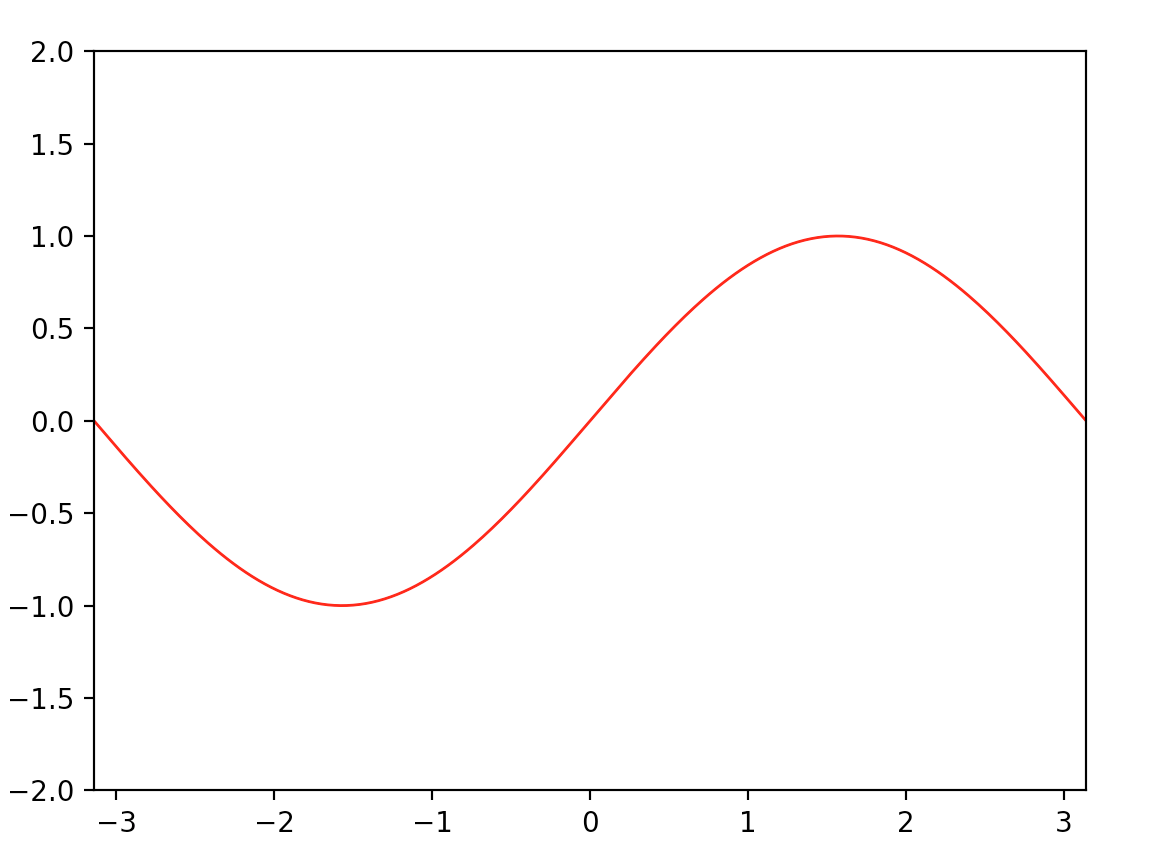### 坐标范围

plt.axis([x_min, x_max, y_min, y_max])

### 坐标间隔设定

import matplotlib.pyplot as plt
import numpy as np

x = np.arange(-2 * math.pi, 2 * math.pi, 0.02)
y = np.sin(x)

plt.axis([-10, 10, -2, 2])

plt.xticks([i * np.pi/2 for i in range(-4, 5)], [str(i * 0.5) + "$\pi$" for i in range(-4, 5)])

plt.yticks([i * 0.5 for i in range(-4, 5)])

plt.xlabel("x")
plt.ylabel("y")

plt.plot(x, y, color="r", linestyle="-", linewidth=1)

plt.show()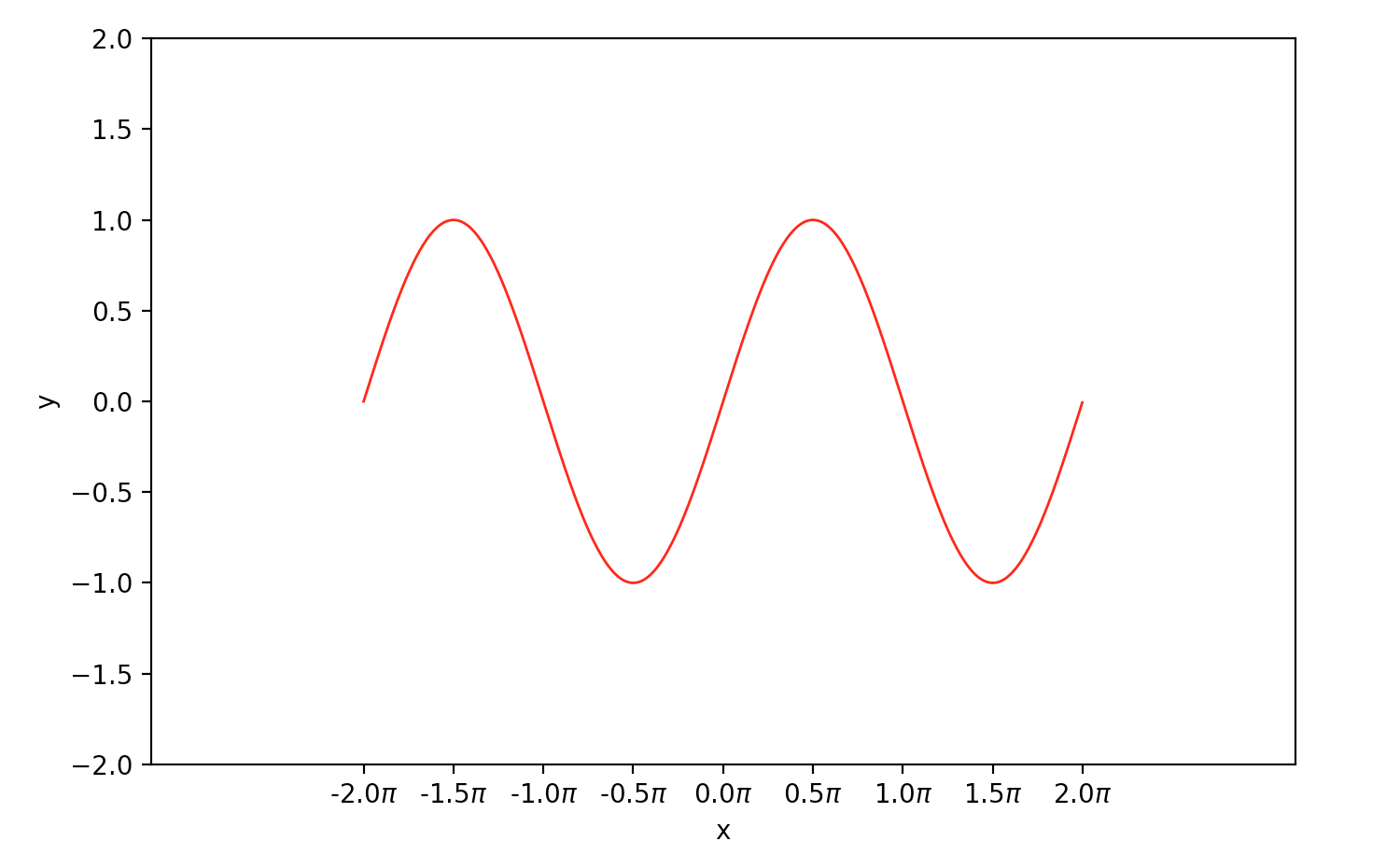## 多图叠加

### 多曲线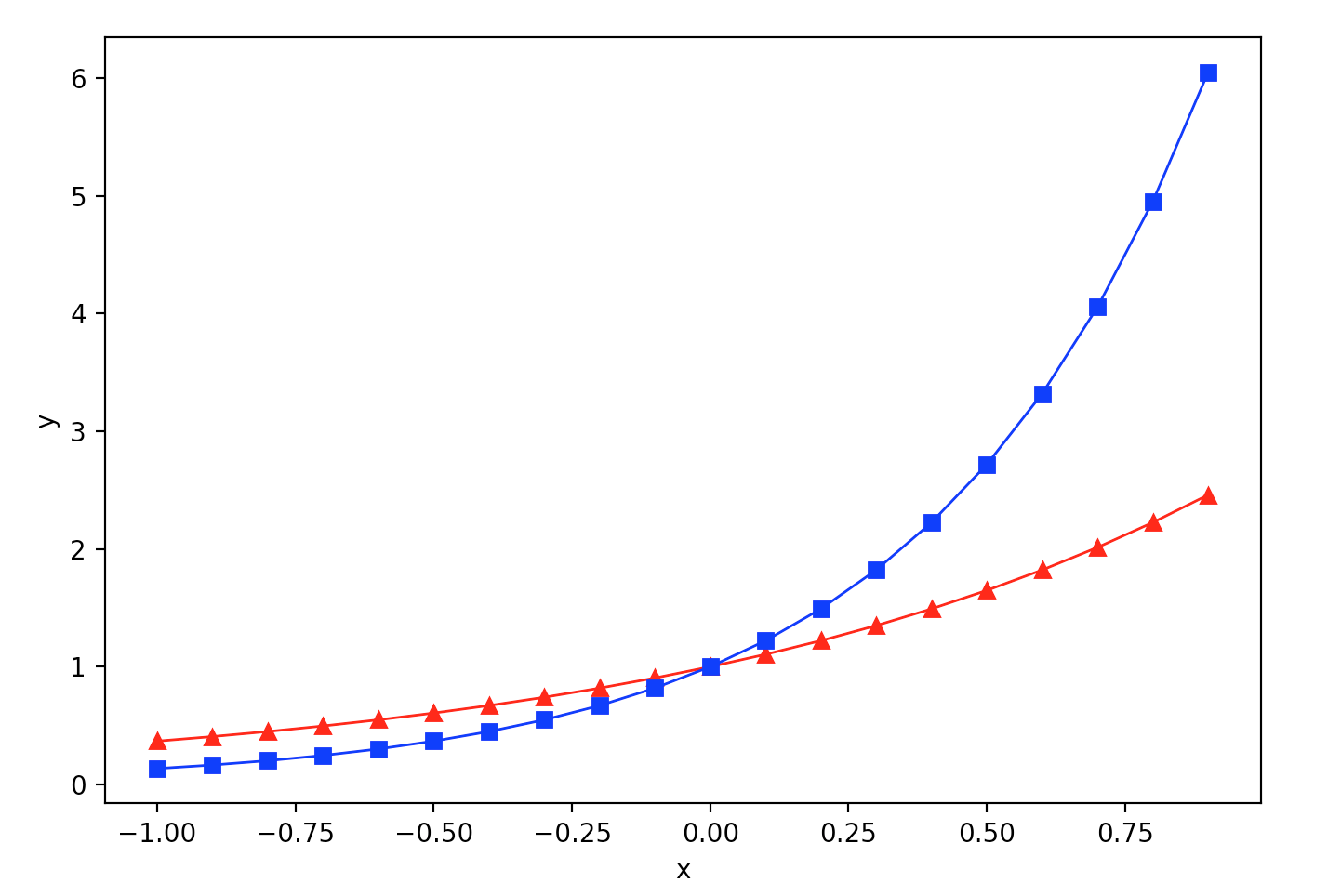import matplotlib.pyplot as plt
import numpy as np

x = np.arange(-1, 1, 0.1)
y1 = np.exp(x)
y2 = np.exp(2 * x)

plt.plot(x, y1, color="r", linestyle="-", marker="^", linewidth=1)

plt.plot(x, y2, color="b", linestyle="-", marker="s", linewidth=1)

plt.xlabel("x")
plt.ylabel("y")

plt.show()

### 多图与多子图

figure()函数可以帮助我们同时处理生成多个图，而subplot()函数则用来实现，在一个大图中，出现多个小的子图。需要注意的是，figure()中的参数为图片序号，一般是按序增加的，这里面还涉及一个当前图的概念，具体可以通过下面的例子来理解；subplot()中的参数一般有3个，分别为行数，列数，以及子图序号。比如，subplot(1, 2, 1)表示这是一个1行，2列布局的图（两个子图，在同一行，分居左右），其中，当前处理的子图是第一个图（也就是左图）。我举个例子：

import matplotlib.pyplot as plt
import numpy as np

x = np.arange(-1, 1, 0.1)

y1 = np.exp(x)
y2 = np.exp(2 * x)
y3 = np.exp(1.5 * x)

plt.figure(1) # 生成第一个图，且当前要处理的图为fig.1
plt.subplot(1, 2, 1) # fig.1是一个一行两列布局的图，且现在画的是左图
plt.plot(x, y1, color="r", linestyle="-", marker="^", linewidth=1) # 画图

plt.xlabel("x")
plt.ylabel("y1")

plt.figure(2) # 生成第二个图，且当前要处理的图为fig.2
plt.plot(x, y2, color="k", linestyle="-", marker="s", linewidth=1) # 画图，fig.2是一张整图，没有子图，默认subplot(1, 1, 1)

plt.xlabel("x")
plt.ylabel("y2")

plt.figure(1) # 当前要处理的图为fig.1，而且当前图是fig.1的左图
plt.subplot(1, 2, 2) # 当前图变为fig.1的右图
plt.plot(x, y3, color="b", linestyle="-", marker="v", linewidth=1)

plt.xlabel("x")
plt.ylabel("y3")

plt.show()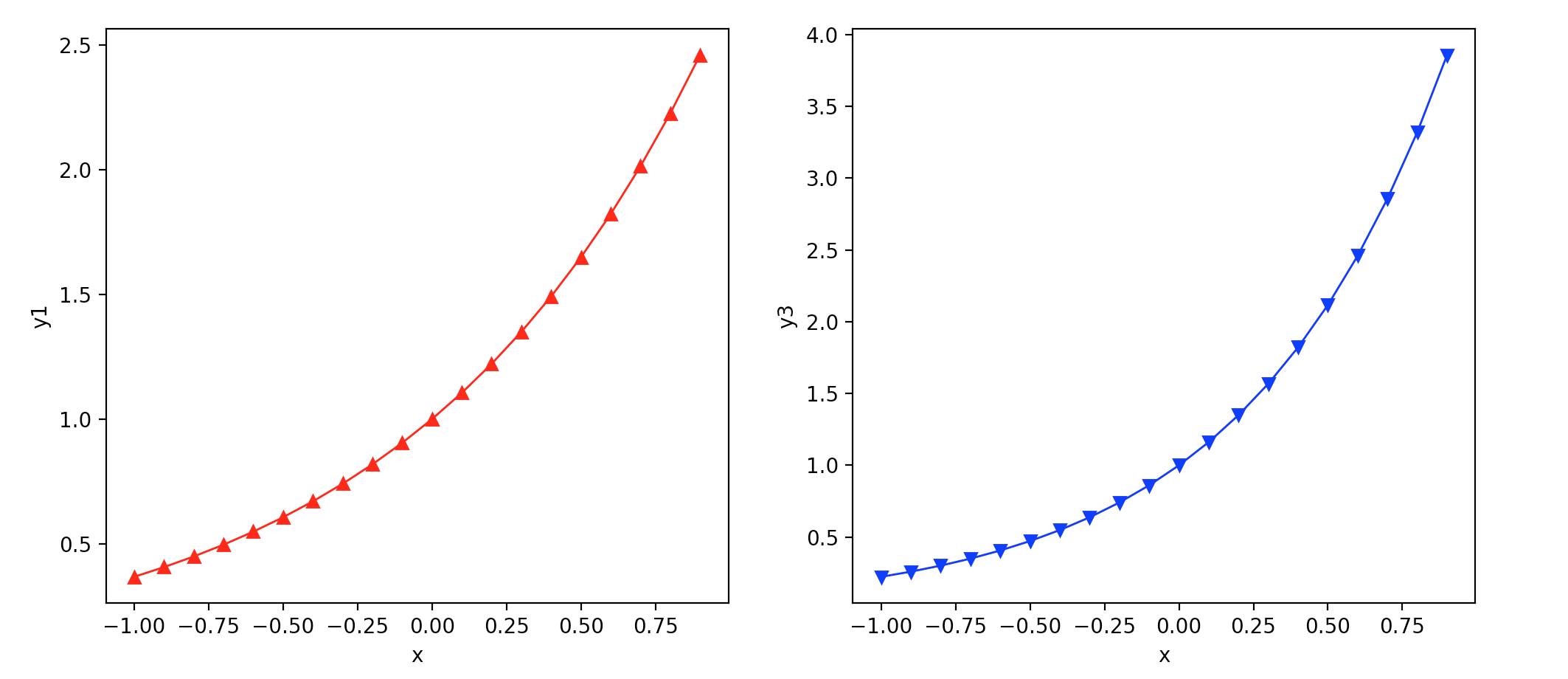plt.subplot(221) # 第一行的左图
plt.subplot(222) # 第一行的右图
plt.subplot(212) # 第二整行
plt.show()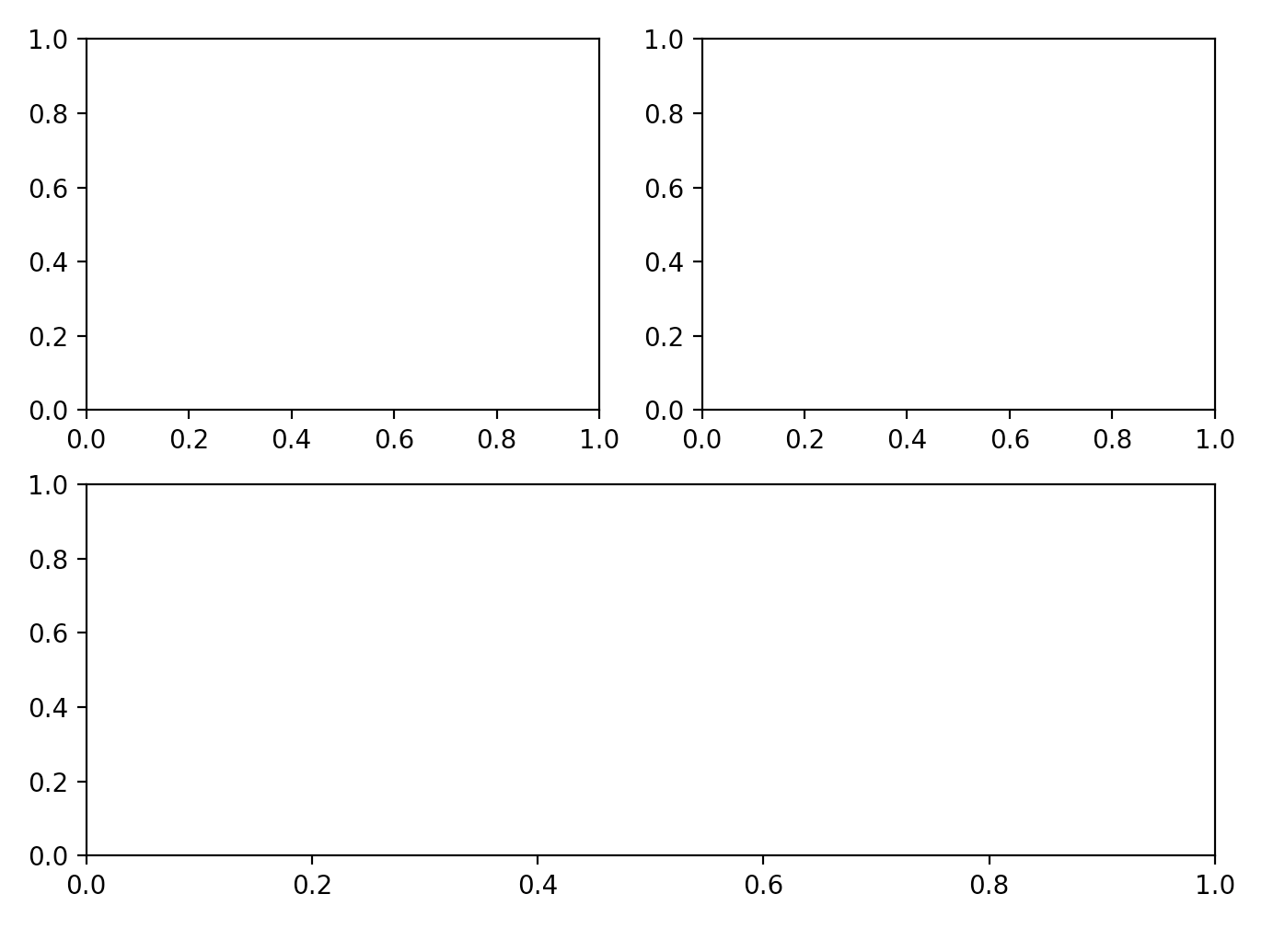## 标题和图例

### 图例 ###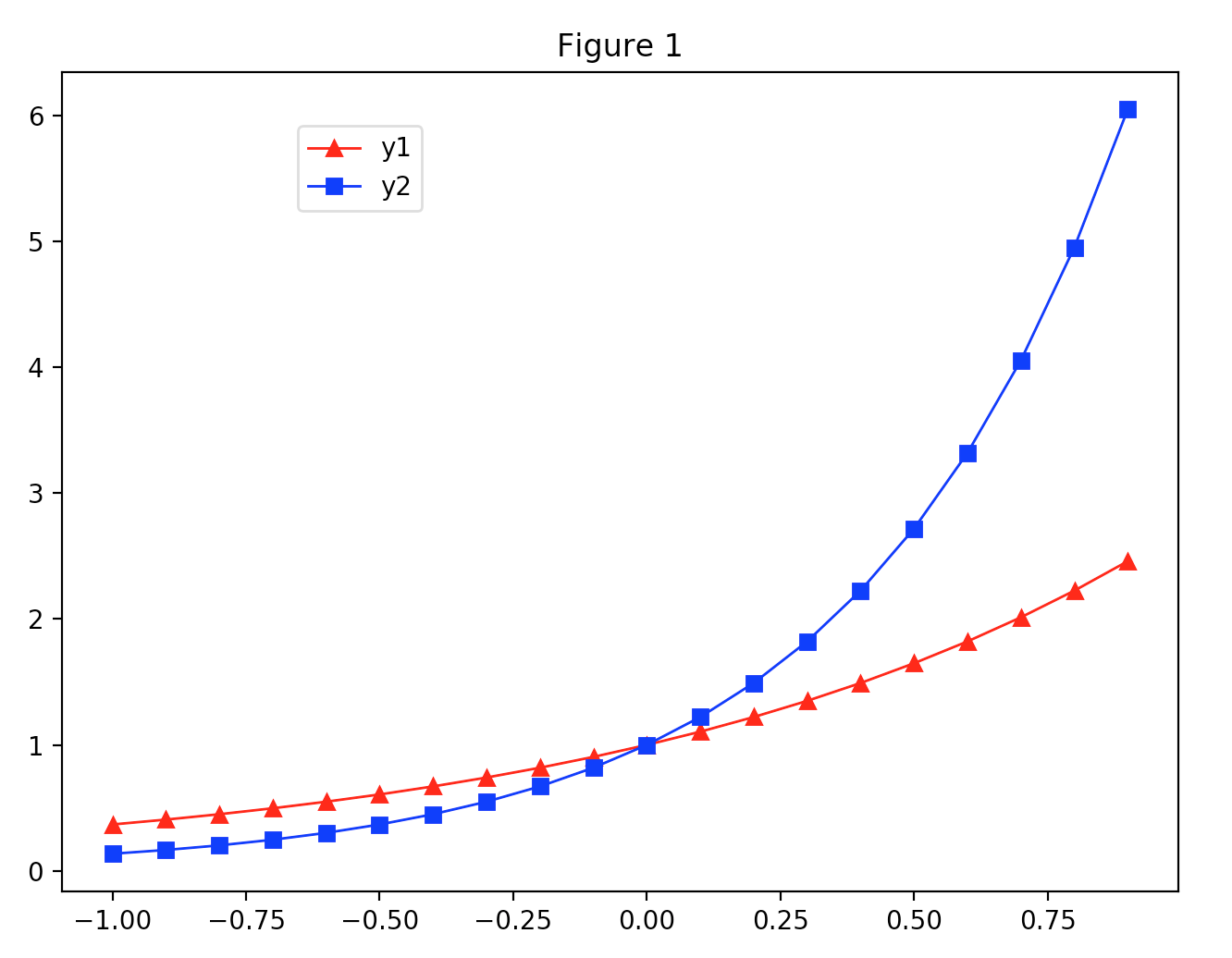import matplotlib.pyplot as plt
import numpy as np

x = np.arange(-1, 1, 0.1)

y1 = np.exp(x)
y2 = np.exp(2 * x)

plt.plot(x, y1, color="r", linestyle="-", marker="^", linewidth=1, label="y1")
plt.plot(x, y2, color="b", linestyle="-", marker="s", linewidth=1, label="y2")

plt.legend(loc='upper left', bbox_to_anchor=(0.2, 0.95))

plt.title("Figure 1")

plt.show()

legend()里面的参数loc表示图例的位置，参数bbox_to_anchor是一个二元组，用来进一步细化图例的位置，其中第一个数值用于控制legend的左右移动，值越大越向右边移动，第二个数值用于控制legend的上下移动，值越大，越向上移动。此外，我们在画两条曲线时，分别为它们添加了标签label，标签的内容也就是最后图例中对应的内容。

## 网格，背景色以及文字注释

### 添加网格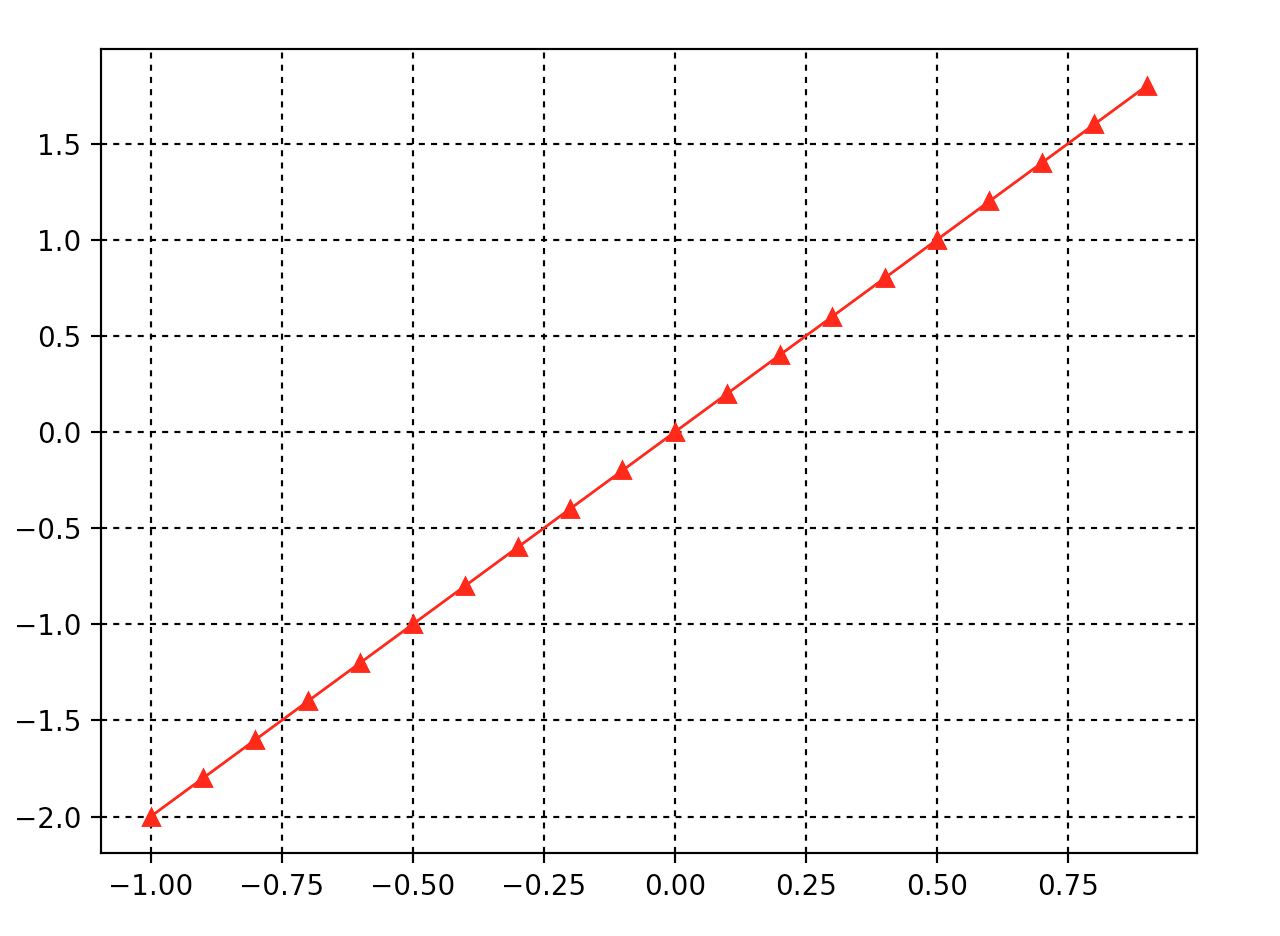import matplotlib.pyplot as plt
import numpy as np

x = np.arange(-1, 1, 0.1)

y = [2 * i for i in x]

plt.plot(x, y, color="r", linestyle="-", marker="^", linewidth=1)

plt.grid(color="k", linestyle=":")
plt.show()

### 背景色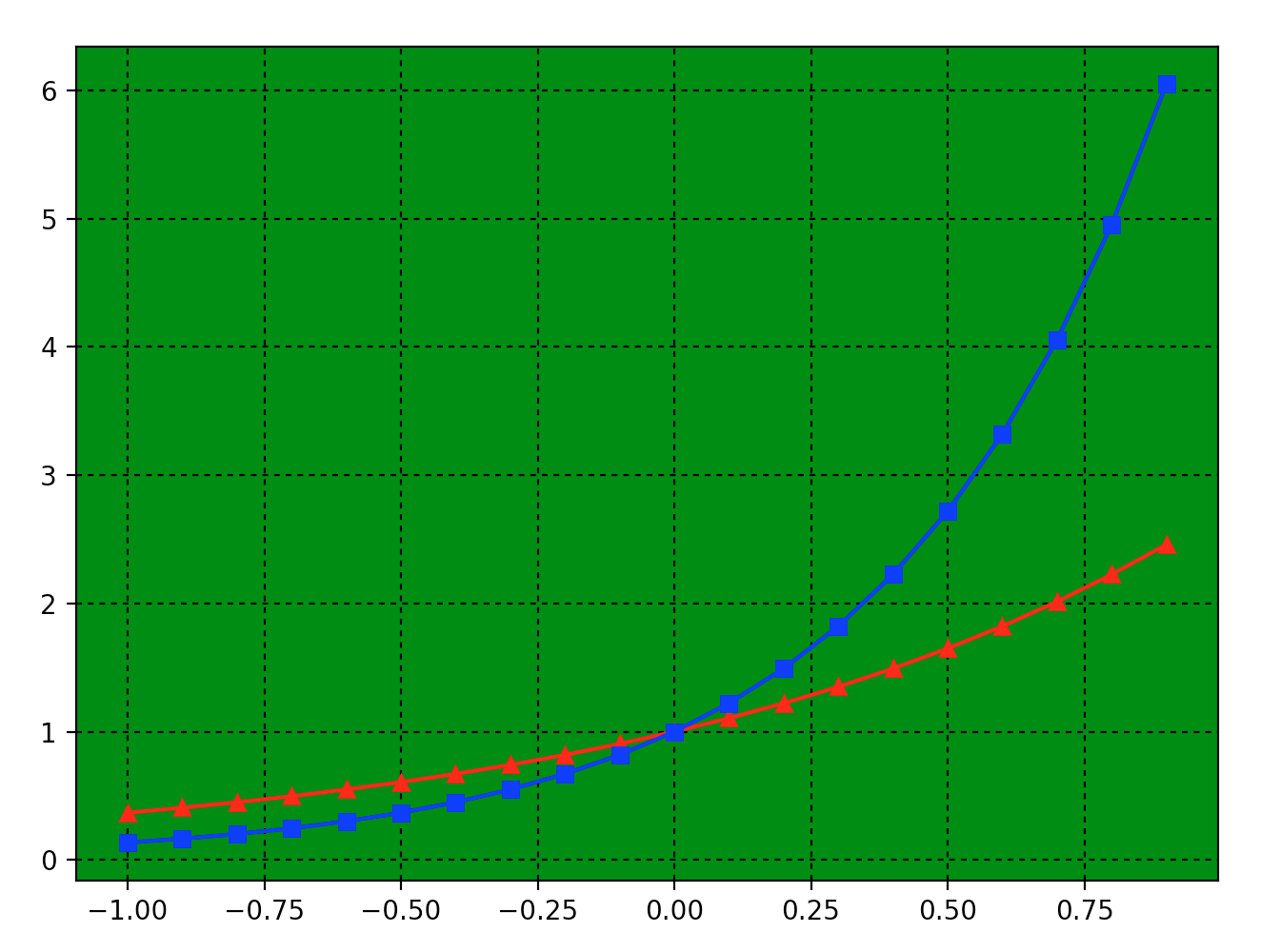import matplotlib.pyplot as plt
import numpy as np

x = np.arange(-1, 1, 0.1)
y1 = np.exp(x)
y2 = np.exp(2 * x)

plt.subplot(facecolor="g")

plt.plot(x, y1, color="r", linestyle="-", marker="^")
plt.plot(x, y2, color="b", linestyle="-", marker="s")

plt.grid(color="k", linestyle=":")
plt.show()

### 文字注释

plt.text(), plt.annotate()是两种向图像添加文本注释的方法。plt.text()可以在图像任意位置添加文字，plt.annotate()则可以帮助我们形象地为曲线中的某一个点添加注释。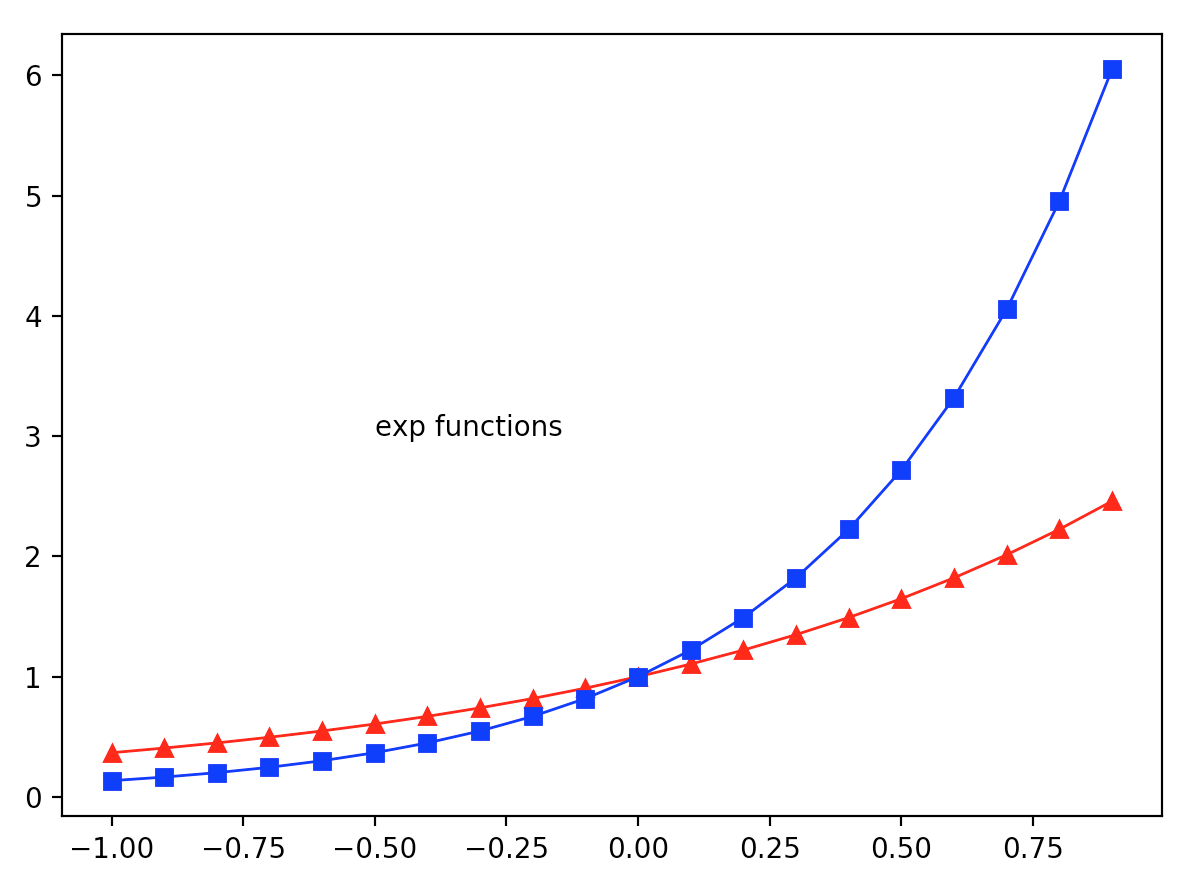import matplotlib.pyplot as plt
import numpy as np

x = np.arange(-1, 1, 0.1)

y1 = np.exp(x)
y2 = np.exp(2 * x)

plt.plot(x, y1, color="r", linestyle="-", marker="^", linewidth=1)
plt.plot(x, y2, color="b", linestyle="-", marker="s", linewidth=1)

plt.text(-0.5, 3, "exp functions", fontsize=10)

plt.show()

plt.text()中前两个参数表示添加文字的坐标位置，最后一个参数是要添加的文本。

plt.annotate()plt.text()类似，代码表示如下：

plt.annotate('A', xy=(0, 1), xytext=(-0.5, 2.5), arrowprops=dict(facecolor='k', headwidth=10, width=2))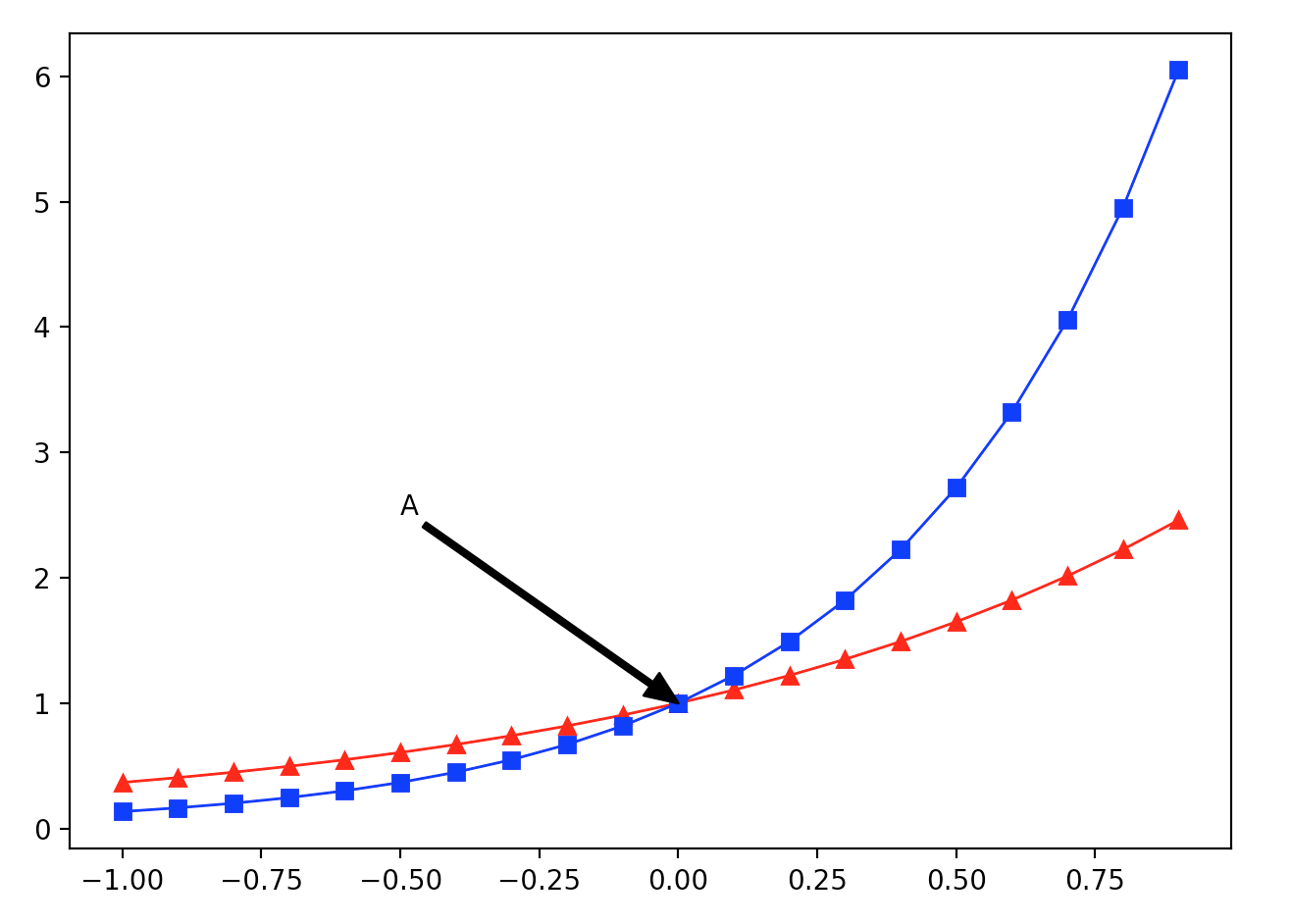02-031万+
06-131278
04-03768
07-04559
04-135971
01-248246
01-303万+
04-244810
08-30379
12-178565
04-291317
04-07767
09-242386

### “相关推荐”对你有帮助么？

•非常没帮助
•没帮助
•一般
•有帮助
•非常有帮助被折叠的  条评论 为什么被折叠?到【灌水乐园】发言guoziqing506

¥2 ¥4 ¥6 ¥10 ¥20余额支付 (余额：-- )扫码支付获取中扫码支付点击重新获取扫码支付1.余额是钱包充值的虚拟货币，按照1:1的比例进行支付金额的抵扣。
2.余额无法直接购买下载，可以购买VIP、C币套餐、付费专栏及课程。余额充值